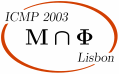ICMP 2003 > Sessions > Operator algebras and quantum information

# Operator algebras and quantum information

Session organized by A. Ocneanu (Penn State), D. Petz (Budapest).

## Talks

 F. Benatti University of Trieste I. Bjelakovic Technische Universität Berlin D. E. Evans Cardiff University Y. Kawahigashi Tokyo C. King Northeast University A.Wassermann CNRS, Luminy

Fabio Benatti
University of Trieste

Quantum dynamical entropies and quantum algorithmic complexities

In the commutative setting, dynamical entropy and algorithmic complexity are related to each other by a theorem of Brudno. Prior to the rapid development of quantum information, several quantum dynamical entropies had been proposed and recently quantum algorithmic complexities have also been put forward.

A study of their interconnections would shed light on the physical content of the various proposals: in this talk, we focus upon the Connes-Narnhofer-Thirring and Alicki-Fannes-Lindblad quantum dynamical entropies and discuss them in the light of information and algorithmic complexity theory.

Igor Bjelakovic
Technische Universität Berlin

Limit theorems for quantum entropies

We present two limit theorems for the von Neumann entropy and the quantum relative entropy. The first one is the Shannon-McMillan-Breiman theorem for ergodic quantum lattice systems. It describes the structure of typical projections of the ergodic states on quasi-local algebras and is basic for coding theorems in quantum information theory. Our second theorem is the ergodic version of the quantum Stein's lemma which was conjectured by Hiai and Petz. The result provides an operational interpretation of the quantum relative entropy as a measure of distinguishability. We indicate some implications of these results.

David E. Evans
Cardiff University

Modular invariants and subfactors

Subfactors provide a natural framework for studying modular invariant partition functions in statistical mechanics and conformal field theory.

We present the general framework of the subfactor theory approach to modular invariants and how it explains the remarkable fusion rule structure of the modular invariant mass matrices in the context of WZW models and quantum doubles particularly of finite groups, and more exotic models. The realisation of defect weights for conjugate modular invariants at the statistical mechanical level is also discussed.

This talk is based on collaborations with Behrend, Boeckenhauer, Kawahigashi and Pinto.

Yasuyuki Kawahigashi
University of Tokyo
Japan

Classification of operator algebraic conformal field theories

We formulate conformal field theory in the setting of algebraic quantum field theory as Haag-Kastler nets of local observable algebras with diffeomorphism covariance on the two-dimensional Minkowski sapce. We then obtain a decomposition of a two-dimensional theory into two chiral theories. We give the first classification result of such chiral theories with representation theoretic invariants. That is, we use the central charge as the first invariant, and if it is less than 1, we obtain a complete classification. Our classification list contains a new net which does not seem to arise from the coset or orbifold constructions. We also explain how to use this to obtain a classification of full two-dimensional theories.

Christopher King
Northeast University

Some applications of matrix inequalities in quantum information theory

Quantum information theory has generated several interesting additivity conjectures, centered around the behavior of products of completely positive maps on matrix algebras. Some special cases of these conjectures have been proved recently, using the Lieb-Thirring inequality and other matrix inequalities. This talk will explain the conjectures and review some of these recent results.

Antony Wassermann
CNRS, Luminy

Operator algebras and positive energy representations

Since the early 1990s it has been known how local von Neumann algebras lead to a tensor product operation on positive energy representations of loop groups. The key was to connect vertex operators with Jones subfactors, Connes fusion and algebraic quantum field theory. This talk reports on two further uses of operator algebras and positive energy representations in elliptic cohomology and statistical mechanics. First we explain how the same local von Neumann algebra yields a "Connes-Jones" 3-cocycle of the underlying group, not only describing spin structure on loop space but also giving a gerbe on the group itself. Then we explain how to make rigorous Baxter's predictions on the spectrum of the corner transfer matrix Hamiltonian, this time connecting q-deformed vertex operators with ground states of C* dynamical systems.

```
```

## Posters

M. Buneci
Univ. Constantin Brancusi of Targu Jiu

The independence of the C*-algebra on the Haar system for groupoids having amenable orbit space

S. Furuichi, K. Yamane
Tokyo

A numerical example both of the optimal decision rule in the quantum channel coding and the concavity of the function appearing in quantum reliability function

M. Keyl
IMP, TU-Braunschweig, Germany

Quantum spin chains as entanglement resources

P. Leroux
IRM, Univ. De Rennes I and CNRS, Campus de Beaulieu, France

From weighted directed graphs to coassociative manifolds

F. Lledó, H. Baumgartel
IPAM, Aachen, Germany and MI, U. Potsdam, Germany

Hilbert C*-systems for C*-algebras with a nontrivial center and duality of compact groups

A. Majewski
ITPA, U. Gdansk, Poland

On quantum dynamical systems - some recent developments

A. Prykarpatsky
UST, Poland

Quantum computing mathematics, holonomic algorithms and their applications

D. Schlingemann
U. Constantin Brancusi, Germany

Algebraic approach to quantum cellular automata

M. Svoboda
Prague

Fine gradings on non-simple Lie algebras: example of O(4,C)

F. Benatti
Trieste

Quantum dynamical entropies and quantum complexities

```
```